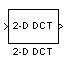2-D DCT

Compute 2-D discrete cosine transform (DCT)

• Library:
• Computer Vision Toolbox / Transforms

•Description

The 2-D DCT block calculates the two-dimensional discrete cosine transform of an image. Suppose f(x,y) is the input image of dimension M-by-N, the equation for the 2-D DCT is

$F\left(m,n\right)=\frac{2}{\sqrt{MN}}C\left(m\right)C\left(n\right)\sum _{x=0}^{M-1}\sum _{y=0}^{N-1}f\left(x,y\right)\mathrm{cos}\frac{\left(2x+1\right)m\pi }{2M}\mathrm{cos}\frac{\left(2y+1\right)n\pi }{2N}$

where $C\left(m\right)=C\left(n\right)=1/\sqrt{2}$ for $m,n=0$ and $C\left(m\right),C\left(n\right)=1$ otherwise.

The number of rows and columns of the input image must be power of 2. You can also use this block to compute 1-D DCT of a vector.

Ports

Input

expand all

Input data, specified as a numeric matrix or a vector. The size of the input data must be power of 2.

Data Types: single | double | int8 | int16 | int32 | int64 | uint8 | uint16 | uint32 | uint64 | Boolean | fixed point

Output

expand all

Output data containing the DCT coefficients, returned as a matrix or vector. The size and the datatype of the output is same as that of the input.

Data Types: single | double | int8 | int16 | int32 | int64 | uint8 | uint16 | uint32 | uint64 | Boolean | fixed point

Parameters

expand all

Main Tab

Use this parameter to specify how the block computes the sine and cosine terms in the DCT algorithm.

• If you select Trigonometric fcn, the block computes the sine and cosine values during the simulation.

• If you select Table lookup, the block computes and stores the trigonometric values before the simulation starts. In this case, the block requires extra memory. Table lookup is the default.

Data Types Tab

Fixed-point operational parameters

Use this parameter to specify the rounding mode for the block to use when the specified data type and scaling cannot exactly represent the result of a fixed-point calculation. See Rounding Modes for more information on the available values.

Note

The sine table values do not obey this parameter; they always round to Nearest.

When you select this parameter, the block saturates the result of its fixed-point operation. When you clear this parameter, the block wraps the result of its fixed-point operation.

For details on saturate and wrap, see Overflow Handling for fixed-point operations.

Note

The sine table values do not obey this parameter; instead, they are always saturated.

Fixed-point data types

Use this parameter to specify the word length of the values of the sine table. The fraction length of the sine table values always equals the word length minus one.

The sine table values do not obey the Rounding mode and Saturate on integer overflow parameters; instead, they are always saturated and rounded to Nearest.

Use this parameter to specify the product output data type. See Fixed-Point Data Types and Multiplication Data Types for illustrations depicting the use of the product output data type in this block.

Use this parameter to specify the accumulator data type. See Fixed-Point Data Types for illustrations depicting the use of the accumulator data type in this block.

Use this parameter to specify the output data type. See Fixed-Point Data Types for illustrations depicting the use of the output data type in this block. When you set this parameter to

• Inherit: Inherit via internal rule, the block calculates the output word length and fraction length automatically. The internal rule first calculates an ideal output word length and fraction length using the following equations:

$W{L}_{idealoutput}=W{L}_{input}+floor\left({\mathrm{log}}_{2}\left(DCTlength-1\right)\right)+1$

$F{L}_{idealoutput}=F{L}_{input}$

Using these ideal results, the internal rule then selects word lengths and fraction lengths that are appropriate for your hardware. For more information, see Inherit via Internal Rule.

Select this parameter to prevent the fixed-point tools from overriding the data types you specify on the block mask. For more information, see fxptdlg (Fixed-Point Designer), a reference page on the Fixed-Point Tool.

Block Characteristics

 Data Types double | fixed point | integer | single Multidimensional Signals no Variable-Size Signals no